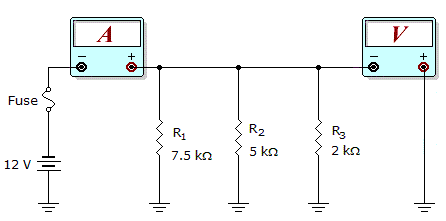# Electronics - Parallel Circuits - Discussion

Discussion Forum : Parallel Circuits - General Questions (Q.No. 11)
11.What would these meter readings indicate about the circuit in the given circuit?

Meter Readings: I = 7.6 mA, V = 12 V

R1 is open.
R2 is open.
The fuse is open.
The circuit is operating normally.
Explanation:
No answer description is available. Let's discuss.
Discussion:
17 comments Page 1 of 2.

CONSIDER THE FOLLOWING:

In Parallel circuit, the current will differ and the voltage will remain same across all the branches. In other words, the voltage across R1, R2 and R3 will be 12V each.

I1= 12/7.5K => 1.6mA
I2= 12/5K => 2.4mA
I3= 12/2K => 6.0mA

In above, the total current 7.6mA will take place correctly when only I1 and I3 present *(since, I1 + I3 = 7.6mA).
Hence, R2 must be discarded/ remove from the circuit. In other words, R2 is open.
(1)

Anomie said:   4 years ago
I=7.6mA so.
~7.6=12V/(7.5K*2K/9.5K).
So R2 is not considered for the meter current so it can a open R2.
(1)

Saish said:   6 years ago
Yes, you are correct. Agree @Addy.
(1)

Well done Saichaitanya and Himanshu Verma.

@ Saichaitanya, Perfect explanation.

Sriram pn said:   1 decade ago
Saichaitanya perfect explanation

Fantastic Himanshu Verma & Saichaitanya ..Great work.

Do the total resistance in the cut by V/I that is 1.578.

Then do the total combination or resist ivory in parallel which resisters combination gives 1.578 ohms and other resistor is open.

Santosh said:   9 years ago
Voltmeter is in parallel connection, so reading is 12V.

Now for resistance calculation which is connected parallel with 2k ohm resistance (R3):

Equivalent resis. R = 12V/7.6mA = (30/19)K ohm.
So, Rx ll R3 = (30/19)k.
or, (1/Rx)+ (1+R3) = 1/(30/19)k.
or, (1/Rx)+ (1/2) = (19/30).
or, (1/Rx) = (19/30)-(1/2).
or, 1/Rx = 4/30.
or, Rx = 30/4 = 7.5k ohm.

i.e., shows R1 is connected & R2 is opened.

Pre said:   7 years ago
Well done @ Himanshu Verma.